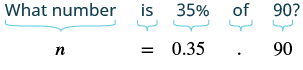## Translating and Solving Basic Percent Equations

### Learning Outcomes

• Translate and solve basic percent equations

We will solve percent equations by using the methods we used to solve equations with fractions or decimals. In the past, you may have solved percent problems by setting them up as proportions. That was the best method available when you did not have the tools of algebra. Now you can translate word sentences into algebraic equations, and then solve the equations.

Remember, percents are fractions, and just like fractions, when finding a percent (or fraction, or portion) of another amount, you multiply.

• the percent, has the percent symbol (%) or the word “percent”
• the amount, the amount is part of the whole
• and the base, the base is the whole amount

Using these parts, we can define equations that will help us answer percent problems.

### The Percent Equation

Percent of the Base is the Amount.

$\text{Percent}\cdot\text{Base}=\text{Amount}$

## Percent of a Whole

We can use this equation to help us solve equations that require us to find the percent of a whole.

For example, if we knew a gas tank held $14$ gallons, and wanted to know how many gallons were in $\frac{1}{4}$ of a tank, we would find $\frac{1}{4}$ of $14$gallons by multiplying:

$\frac{1}{4}\,\cdot \,14=\frac{1}{4}\,\cdot \,\frac{14}{1}=\frac{14}{4}=3\frac{2}{4}=3\frac{1}{2}\,\,\,\text{gallons}$

Likewise, if we wanted to find  $25\%$ of $14$ gallons, we could find this by multiplying, but first we would need to convert the 25% to a decimal:

$25\%\,\,\text{of}\,\,14\,\,\,\text{gallons}=0.25\,\cdot \,14=3.5\,\,\,\text{gallons}$

### Finding a Percent of a Whole

To find a percent of a whole,

• Write the percent as a decimal by moving the decimal two places to the left
• Then multiply the percent by the whole amount

### Example

What is $15$% of \$$200$?

The following video contains an example that is similar to the one above.

In the examples below, the unknown is represented by the letter n. The unknown can be represented by any letter or a box □, question mark, or even a smiley face :)

In the next examples, we will find the amount. We must be sure to change the given percent to a decimal when we translate the words into an equation.

### example

What number is $\text{35%}$ of $90?$

Solution

 Translate into algebra. Let $n=$ the number.Multiply. $n=31.5$ $31.5$ is $35\text{%}$ of $90$

### example

$\text{125%}$ of $28$ is what number?

### try it

The video that follows shows how to use the percent equation to find the amount in a percent equation when the percent is greater than $100\%$.

https://youtu.be/dO3AaW_c9s0he

## Solve for the Base

In the next examples, we are asked to find the base.

Once you have an equation, you can solve it and find the unknown value. For example, to solve  $20\%\cdot{n}=30$ you can divide $30$ by $20\%$ to find the unknown:

$20\%\cdot{n}=30$

You can solve this by writing the percent as a decimal or fraction and then dividing.

$20\%\cdot{n}=30$

$n=30\div20\%=30\div0.20=150$

### Example

Write an equation that represents the following problem.

$30$ is $20\%$ of what number?

### example

Translate and solve: $36$ is $\text{75%}$ of what number?

### example

$\text{6.5%}$ of what number is $\text{\1.17}$?

### try it

In the following video we show another example of how to find the base or whole given percent and amount.

## Solve for the Percent

In the next examples, we will solve for the percent.

### Example

What percent of $72$ is $9$?

You can estimate to see if the answer is reasonable. Use $10\%$ and $20\%$, numbers close to $12.5\%$, to see if they get you close to the answer.

$10\%$ of $72$ = $0.1$ · $72$ = $7.2$

$20\%$ of $72$ = $0.2$ · $72$ = $14.4$

Notice that $9$ is between $7.2$ and $14.4$, so $12.5\%$ is reasonable since it is between  $10\%$ and $20\%$.

### example

What percent of $36$ is $9?$

### example

$144$ is what percent of $96?$

### try it

In the next video we show another example of how to find the percent given amount and the base.

## Contribute!

Did you have an idea for improving this content? We’d love your input.